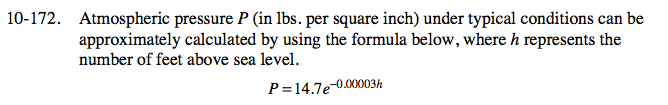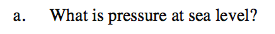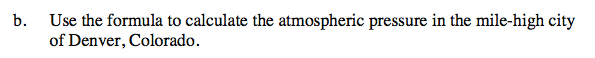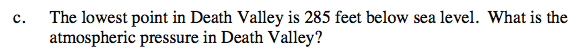Home > CCA2 > Chapter 10 > Lesson 10.3.2 > Problem10-172

10-172.
1. Atmospheric pressure P (in lbs per square inch) under typical conditions can be approximately calculated by using the formula below, where h represents the number of feet above sea level. Homework Help ✎

2. P = 14.7e−0.00003h

1. What is pressure at sea level?

2. Use the formula to calculate the atmospheric pressure in the mile-high city of Denver, Colorado.

3. The lowest point in Death Valley is 285 feet below sea level. What is the atmospheric pressure in Death Valley?P = 14.7e−0.00003(0)

14.7 psiP = 14.7e−0.00003(5280)P = 14.7e−0.00003(−285)